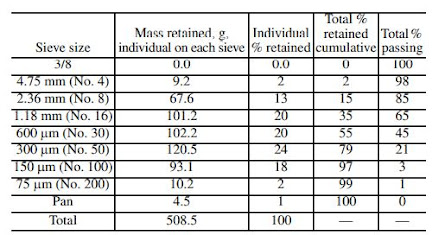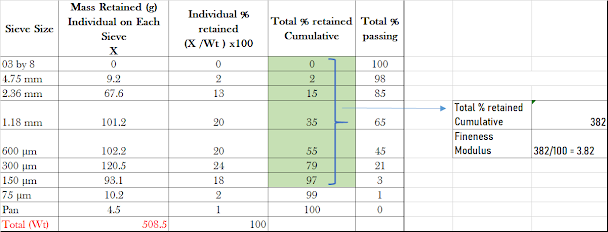# Sieve Analysis of Aggregates - ASTM Standard

Grading refers to the distribution of different particle sizes in an aggregate. The grading is determined as per American standards, ASTM C 136, "Sieve or Screen Analysis of Fine and Coarse Aggregates." The process is called sieve analysis. Fineness modulus (FM) is a numerical index used to determine the extent of fineness of aggregates.Sieve Analysis of Aggregates - Grading Aggregates & Fineness Modulus

In the grading process or sieve analysis, sample of aggregate is shaken through a series of wire-cloth sieves with square openings, that is nested one over the other in the decreasing order of sieve size. Largest sieve size is placed top and at bottom the sieve with small size and pan at bottom to receive particles that pass through the smallest sieve.

The commonly used sieve sizes for concrete aggregates are given in Table-1 below for both coarse sieves and fine sieves.

### Grading Coarse and Fine Aggregates

• Coarse and fine aggregates are sieved separately.
• That portion of aggregates that pass through a 4.75 mm sieve ( From Table-1, No: 4 Sieve) and that retains on the 75 μm sieve ( No.200 sieve) is called as fine aggregates.
• Aggregates larger than 4.75 mm sieve size are coarse aggregates. Coarse aggregates are available in several different size groups like 19 to 4.75 mm (3/4 in. to No. 4), or 37.5 to 19 mm (1-1/2 to 3/4 in.).
• The number and size of sieves that is selected for conducting sieve analysis is dependent on the particle size present in the sample and type of grading required.

### Sieve Analysis of Coarse-Grained Soils

After sieving the sample of aggregates through the select series of sieves, the mass of material retained on each sieve and the last pan is measured using a balance that is accurate to 0.1%.

The results are recorded in a tabular form ( Fig.2) that shows the:

1. Quantity of aggregates retained on each sieve
goog_583263229Individual percentage of aggregates retained (Wr) and passing (Wp) through the sieve. Individual percent retained is the percentage of material contained between successive sieves, recorded to the nearest whole percent. It is calculated by dividing the mass retained on each sieve (and passing the sieve above) by the sum of the masses retained on each sieve and the pan and multiplying by 100.                                                                   W = ( X + Wt) x 100;                                                                          W = Individual percent retained, X is the weight of the oven dry aggregate retained on the individual sieve or pan, and Wt is the total mass of dry sample ( Sum of mass retained on sieves and the pan).                                                                                For eg, in the figure-2 below, considering the sieve 4.75 mm No.4 ,                                                                              The mass retained on 4.75 mm sieve = X =9.2 g;                                                           The total weight of sample = 508.5;                                Then, W1 ( Individual percent retained in sieve No.4) = (X/Wt)x100                                                                                                                    = (9.2/508.5)x 100 = 2 %
1. Total percent of retained cumulative ( cumulative of retained is taken - i.e. 2, (2+13=15), (15+20 =35), (35 +20 =55) so on.)
2. Total percent of the whole sample passing or retaining through each sieve. The total percent passing is calculated by subtracting the total (cumulative) percent retained from 100. In the table below, for sieve 4.75 mm, total percent passing is 100-2 =98, for 2.36 mm sieve it is 100 - 15 =85, so on.Results of Sieve Analysis Presented in a Tabular Form

Important Points To Remember:
1. The total mass retained may differ from the original, as sieving process may result in loss or gain of aggregate particle or due to round-off errors.
2. As each mass is measured within 0.1% of the total mass, maximum difference must not exceed 0.1% times the number of mass determinations. For example, in the above  test result, 7 mass determinations were made, so the difference should not exceed 0.7 %.
3. In the above example, the mass of original sample was 510.5 g. From the figure-2, the total mass retained after test was 508.5g, which give a difference from original sample by 2 g, i.e. 0.4% difference, which is less than 0.7 % mentioned before, which is accepted.
4. If the difference is too large, a check would have been made for possible errors in mass determination, calculation, accidental loss due to spillage, or material stuck in the sieve openings.
5. Normally, the sieve analysis calculations are done to the nearest 0.1% and then reported to the nearest 1%, except for the percent passing the No. 200 sieve, which is reported to the nearest 0.1%.
6. The total mass of the material after sieving should check closely with the original mass of the sample placed on the sieves. If the amounts differ by more than 0.3%, based on the original dry sample mass, the results should not be used for acceptance purposes.

To measure the accurate quantity of aggregate material finer than 75 μm, the specimen is washed as per ASTM C 117. This is performed before sieving or on another sample.

### Grain Size Distribution of Coarse-Grained Soils

Grading charts are used to represent the results obtained after the sieve analysis graphically.
1. In the vertical axis or Y-Axis, the percent passing through the sieve is plotted
2. In the X-Axis, the sieve sizes adopted for the test are plotted
3. The upper and lower limits that is specified for allowable percentage of material passing through the sieve may also be included in grading chart.Typical grading chart. Dashed lines indicate limits specified in ASTM C 33 for fine aggregates and for 25.0 mm (1 in.) coarse aggregate.

To evaluate consistency of grading the individual size fractions of a coarse aggregate and fine aggregate, the grading charts are plotted separately, so that any gaps or excess amounts of particle sizes can be analyzed.

### Fineness Modulus

Fineness modulus (FM) is a numerical index obtained from the sieve analysis test result. The FM is the sum of the total percentages coarser than each of a specified series of sieves, divided by 100. In the figure-3, the sum of total cumulative percentage retained divided by 100 gives the fineness modulus.Calculation of Fineness Modulus of Aggregates

Although the FM is most commonly computed for fine aggregates, the FM of coarse aggregate is needed for some proportioning methods. It is calculated in the same manner, while taking care to exclude sieves that are not specified in the definition (for example, 25.0 and 12.5 mm [l and 1/2 in.] sieves) and to include all of the specified finer sieves.

Important Points To Remember:

1. Coarser the aggregate, higher is the fineness modulus.
2. FM generally ranges between 2.3 to 3.1 for fine aggregates used in concrete as per ASTM C 33.

1.2.